# Free Thin Square PlateThis test lets you check analysis results for a free thin square plate, in the context of a Free Frequency Case. You will use 2D meshes.

This test validates the following attributes:

• Rigid body modes (3 modes)
• Repeated eigen values
• Cinematically incomplete suppressions.Reference:

NAFEMS-Glasgow, BENCHMARK newsletter, April 1989, p.17.

##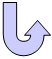Specifications

### Geometry Specifications

 Length: L = 10000 mm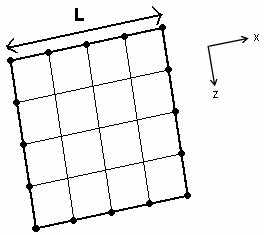Thickness: th = 50 mm

### Analysis Specifications

 Young Modulus (material): E = 200 GPa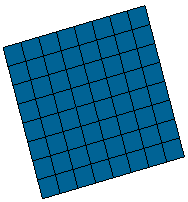Poisson's Ratio (material): ν = 0.3 Density: ρ = 8000 kg/m3 Mesh Specifications: 8 x 8 elements mesh for linear triangle (TR3) and linear quadrangle elements (QD4) 4 x 4 elements mesh for parabolic triangle (TR6) and parabolic quadrangle elements (QD8) Restraints (User-defined): The structure is free, hence the boundary conditions are: Ty = Tz = Rx = 0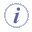The Frequency case must be computed in Lanczos method with Shift Auto.

##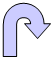Results

The computed results present the frequency of the six modes after the three rigid body modes.

The normalized results are the computed results divided by the reference solution.

 Mode Reference value [Hz] Values Linear triangle (TR3) Linear quadrangle (QD4) Parabolic triangle (TR6) Parabolic quadrangle (QD8)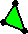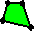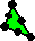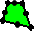Computed  results [Hz] Normalized  results Computed  results [Hz] Normalized  results Computed  results [Hz] Normalized  results Computed  results [Hz] Normalized  results 4 1.622 1.614 0.995 1.624 1.001 1.584 0.977 1.622 1.000 5 2.360 2.379 1.008 2.389 1.012 2.376 1.007 2.367 1.003 6 2.922 2.961 1.013 2.980 1.020 2.950 1.010 2.935 1.004 7 4.233 4.229 0.999 4.252 1.004 4.135 0.977 4.174 0.986 8 4.233 4.231 1.000 4.252 1.004 4.149 0.980 4.174 0.986 9 7.416 7.653 1.032 7.793 1.051 7.439 1.003 7.550 1.018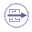To Perform  the Test

The Free_Thin_Square_Plate_QD4.CATAnalysis document presents a complete analysis of this case, computed with a mesh formed of linear quadrangle elements (QD4).

To compute the case with other elements (QD8, TR3 and TR6), proceed as follow:

1. Open the CATAnalysis document.

2. In the Advanced Meshing Tools workbench, replace the mesh specifications as indicated above.

3. In the Generative Structural Analysis workbench, compute the case.